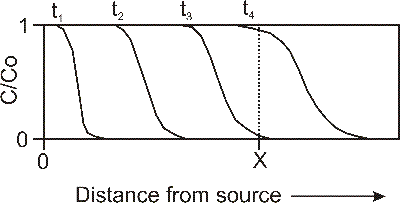## Calculator 5 - Transport 1-D (Ogata Banks)

###The Ogata-Banks calculator solves the 1D advection dispersion equation using the Ogata-Banks solution.  The input for the calculator includes a time of interest, location of interest (distance from the source) and a source concentration, in addition to the transport properties of velocity, dispersion and retardation.  In this figure, you can see the breakthrough curves for a contaminant along a 1D flowpath from the source, at four different times.  If distance 'X' had been chosen at time 3, the calculator would return a very low concentration (as seen by where the t3 breakthrough meets the dotted line indicating point 'X').  If time 4 had been chosen, the concentration would be significantly higher (as seen by where the t4 breakthrough meets the dotted line).OGATA-BANKS CALCULATOR

Input

t (time of interest):

Co (constant source concentration):

v (groundwater velocity):

D (dispersion):

R (retardation factor):

**KEEP UNITS CONSISTENT**

Results:

Concentration*:

* Concentration will have same units as CoExample (from Fetter, 1994):

A landfill is leaking leachate with a chloride concentration of 725 mg/L, which enters an aquifer with the following properties:

Hydraulic conductivity = 3.0 x 10-3 cm/s

Effective porosity = 0.23

Dispersion = 2.4 x 10-7 m2/s

Compute the concentration of chloride in 1 y at a distance 15m from the point where the leachate entered the groundwater.  Chloride has a retardation factor of 1.0.

Solution:

Determine the groundwater velocity:

vx = K(dh/dl)/ne (click to go to velocity calculator)

vx = 3.0 x 10-5 m/s x 0.0020/0.23 = 2.6 x 10-7 m/s

Using all this data, and converting the time from 1 year into 3.15 x 107 seconds, the concentration at 15 m would be approximately 30 mg/L.

Reference

Fetter, C.W. (1994).  Applied Hydrogeology, Third Edition.  Published by Prentice-Hall Inc.,  New Jersey, USA.